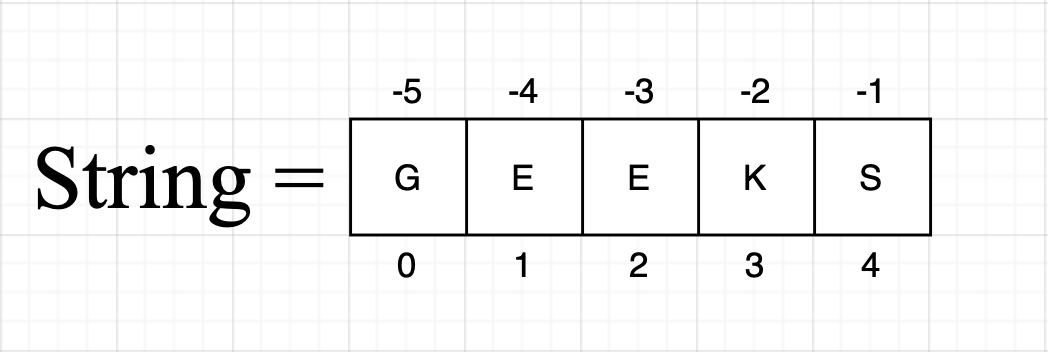Open In App

# Python Program to Find Sum of First and Last Digit

Given a positive integer N(at least contain two digits). The task is to write a Python program to add the first and last digits of the given number N.

Examples:

```Input: N = 1247
Output: 8

Explanation: First digit is 1 and Last digit is 7.
So, addition of these two (1 + 7) is equal to 8.```
```Input: N = 73
Output: 10```

### Method 1: String implementation

• Take input in the form of String or typecast given input in String.
• Now pick the 0th index of the String and typecast it into Integer and store it in a variable.
• The same thing with the -1st index and also store in another variable.
• Now add these two variables and
• print them as an Output.

Note:  We can access the first element of String using string and the last element of String using string[-1].String Representation

## Python3

 `# We have a number``number ``=` `1247` `# We are type casting it in string``number ``=` `str``(number)` `# Storing first and last digit in a variable``# after type casting into Integer.``first_digit ``=` `int``(number[``0``])``last_digit ``=` `int``(number[``-``1``])` `# Adding these two variables``addition ``=` `first_digit ``+` `last_digit` `# Display our output``print``(``'Addition of first and last digit of the number is'``,``      ``addition)`

Output

`Addition of first and last digit of the number is 8`

Output:

Addition of first and last digit of the number is 8

Time complexity: O(1) since performing constant operations

Auxiliary Space: O(1) since using constant space for variables

### Method 2: Solve it using an integer

• We have given a positive Integer.
• After dividing by 10, store the remainder in a result variable.
• Continue the loop until the number becomes less than 9.
• Each time in the loop, divide the number by 10(integer division).
• After the end of the loop.
• Add the number in the result variable.
• Display the result variable as the output.

Note: Whenever we divide any number with 10, we get the last digit as the remainder. If we divide any number with 100, we get the last two-digit as the remainder.

## Python3

 `# We have a number.``number ``=` `1247` `# Assigning last digit of the number in res``# variable.``res ``=` `number ``%` `10` `# Now, continue a loop until``# the number becomes less than 9.``while` `number > ``9``:` `    ``# integer division of the number and reassigning``    ``# it.``    ``number ``=` `number ``/``/` `10` `# Here, our number only contain one digit.``# So, add this number in res variable.``res ``+``=` `number` `# Now, display our output``print``(``'Addition of first and last digit of number is'``, res)`

Output

`Addition of first and last digit of number is 8`

Output:

`Addition of first and last digit of the number is 8`

Time Complexity: O(n), where n is how many digits are there in the given number.

Auxiliary Space: O(1) since using constant space for variables

### String Conversion and Digit Extraction Method

Steps:

1. Take the input number as input from the user.
2. Convert the input number to a string using the str() function.
3. Extract the first digit by taking the first character of the string using the indexing operator.
4. Extract the last digit by taking the last character of the string using the indexing operator.
5. Convert the first and last digit from string to integer using the int() function.
6. Add the first and last digit.
7. Return the sum.

## Python3

 `def` `sum_of_first_and_last_digit(n):``    ``# convert the input number to a string``    ``str_n ``=` `str``(n)``    ` `    ``# extract the first and last digit``    ``first_digit ``=` `int``(str_n[``0``])``    ``last_digit ``=` `int``(str_n[``-``1``])``    ` `    ``# add the first and last digit``    ``sum` `=` `first_digit ``+` `last_digit``    ` `    ``# return the sum``    ``return` `sum``#Example``n ``=` `1247``result ``=` `sum_of_first_and_last_digit(n)``print``(result)`

Output

`8`

Time Complexity: O(1)

Auxiliary Space: O(1)

### Method: Using Mathematical Formula

Steps:

1. Take the input integer as N.
2. Find the number of digits in N by taking the logarithm of N to the base 10 and adding 1 to it.
3. Find the first digit of N by dividing N by 10 raised to (number of digits – 1).
4. Find the last digit of N by taking the modulus of N with 10.
5. Add the first and last digits to get the sum.
6. Print the output.

## Python3

 `import` `math` `def` `sum_of_first_and_last_digit(N):``    ``# find the number of digits in N``    ``num_digits ``=` `int``(math.log10(N)) ``+` `1``    ` `    ``# find the first digit of N``    ``first_digit ``=` `N ``/``/` `(``10``*``*``(num_digits``-``1``))``    ` `    ``# find the last digit of N``    ``last_digit ``=` `N ``%` `10``    ` `    ``# add the first and last digits``    ``# to get the sum``    ``sum` `=` `first_digit ``+` `last_digit``    ` `    ``# return the sum``    ``return` `sum` `# Driver Code` `# Sample Input 1``N ``=` `1247` `# Calling function and printing output``print``(sum_of_first_and_last_digit(N))` `# Sample Input 2``N ``=` `73` `# Calling function and printing output``print``(sum_of_first_and_last_digit(N))`

Output

```8
10```

Time Complexity: O(1)
Auxiliary Space: O(1)

#### Approach#5:using lambda

In this code, we take the input number from the user, convert it to an integer, and then use an anonymous lambda function to find the sum of the first and last digits of the number. The lambda function calculates the last digit using the modulus operator and the first digit using string manipulation.

#### Algorithm

• Take the input number from the user using the input() function.
• Convert the input string to an integer using the int() function.
• Define an anonymous lambda function that takes an integer argument x and calculates the sum of the first and last digits of x using the modulus operator and string manipulation.
• Pass the input number to the lambda function using the function call syntax (sum(n)).
• Print the sum of the first and last digits of the number using the print() function.

## Python3

 `n ``=` `1247`  `def` `sum``(x): ``return` `x ``%` `10` `+` `int``(``str``(x)[``0``])`  `print``(``"Sum of first and last digit:"``, ``sum``(n))`

Output

`Sum of first and last digit: 8`

Time complexity: O(1)
Auxiliary Space: O(1)